SPSS TUTORIALS BASICS ANOVA REGRESSION FACTOR CORRELATION

# SPSS – Missing Values for String Variables

Can you set missing values for string variables in SPSS? Short answer: yes. Sadly, however, this doesn't work as it should. This tutorial walks you through some problems and fixes.

## Example Data

All examples in this tutorial use staff.sav, partly shown below. We'll run some basic operations on Job Type (a string variable) and Marital Status (a numeric variable) in parallel.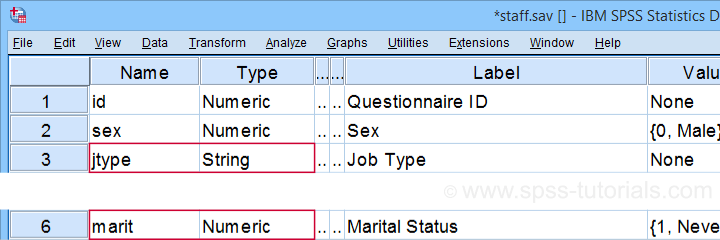Let's first create basic some frequency distributions for both variables by running the syntax below.

*Minimal frequency table for numeric and string variable.

frequencies marit jtype.

## Result

Let's take a quick look at the frequency distribution for our string variable shown below.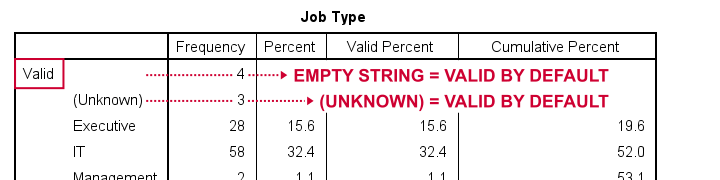By default, all values in a string variable are valid (not missing), including an empty string value of zero characters.

## Setting Missing Values for String Variables

Now, let's suppose we'd like to set the empty string value and (Unknown) as user missing values. Experienced SPSS users may think that running missing values jtype ('','(Unknown)'). should do the job. Sadly, SPSS simply responds with a rather puzzling warning:

>Warning # 4812 in column 26. Text: (Unknown)
>The missing value is specified more than once.

First off, this warning is nonsensical: we didn't specify any missing value more than once. And even if we did. So what?

Second, the warning doesn't tell us the real problem. We'll find it out if we run missing values jtype ('(Unknown)'). This triggers the warning below, which tells us that our user missing string value is simply too long.

>Error # 4809 in column 23. Text: (Unknown)
>The missing value exceeds 8 bytes.
>Execution of this command stops.

A quick workaround for this problem is to simply RECODE '(Unknown)' into something shorter such as 'NA' (short for ‘Not Available”). The syntax below does just that. It then sets user missing values for Job Type and reruns the frequency tables.

*Convert (Unknown) into shorter string value.

recode jtype ('(Unknown)' = 'NA').

*Set 2 user missing values for string variable.

missing values jtype ('','NA').

*Quick check.

frequencies marit jtype.

## Result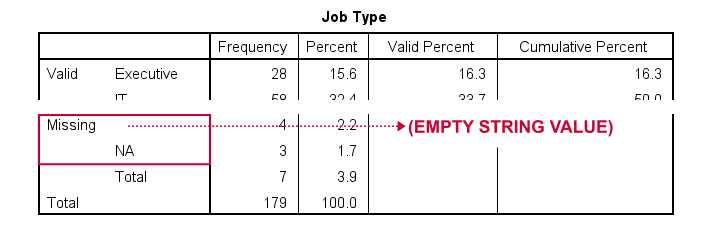At this point, everything seems fine: both the empty string value and 'NA' are listed in the missing values section in our frequency table.

## Missing String Values and MEANS

When running basic MEANS tables, user missing string values are treated as if they were valid. The syntax below demonstrates the problem:

*Basic MEANS command for numeric by numeric and string variable.

means salary by marit jtype
/statistics anova.

## Result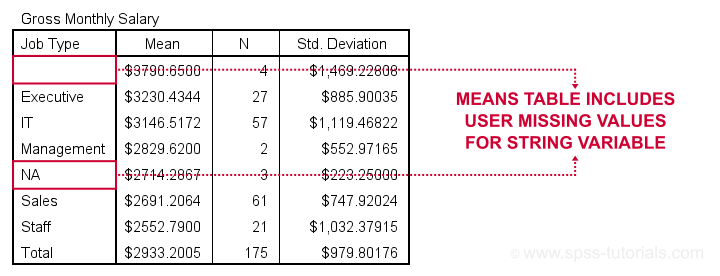Note that user missing values are excluded in the first means table but included in the second means table. As this doesn't make sense and is not documented, I strongly suspect that this is a bug in SPSS.

Let's now try some CROSSTABS.

## Missing String Values and CROSSTABS

We'll now run two basic contingency tables from the syntax below.

*Basic CROSSTABS for numeric by numeric and string variable.

crosstabs marit jtype by sex.

## Result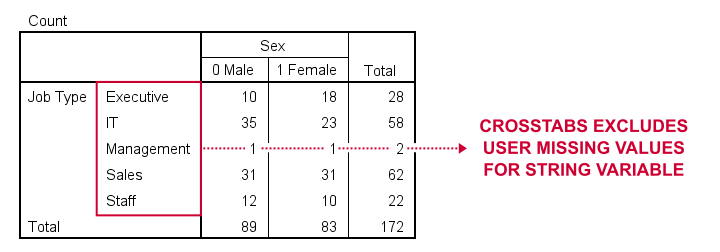Basic conclusion: both tables fully exclude user missing values for our string and our numeric variables. This suggests that the aforementioned issue is restricted to MEANS. Unfortunately, however, there's more trouble...

## Missing String Values and COMPUTE

A nice way to dichotomize variables is a single line COMPUTE as in compute marr = (marit = 2). In this syntax, `(marit = 2)` is a condition that may be false for some cases and true for others. SPSS returns 0 and 1 to represent false and true and hence creates a nice dummy variable.

Now, if marit is (system or user) missing, SPSS doesn't know if the condition is true and hence returns a system missing value on our new variable. Sadly, this doesn't work for string variables as demonstrated below.

*Dichotomize numeric variable (correct result).

compute marr = (marit = 2).

variable labels marr 'Currently married (yes/no)'.

frequencies marr.

*Dichotomize string variable (incorrect result).

compute it = (jtype = 'IT').

variable labels it 'Job type IT (yes/no)'.

frequencies it.

A workaround for this problem is using IF. This shouldn't be necessary but it does circumvent the problem.

*Remove "it" before recomputing it correctly.

delete variables it.

*Dichotomize string variable (correct result).

if(not missing(jtype)) it = (jtype = 'IT').

variable labels it 'Job type IT (yes/no)'.

frequencies it.

## Result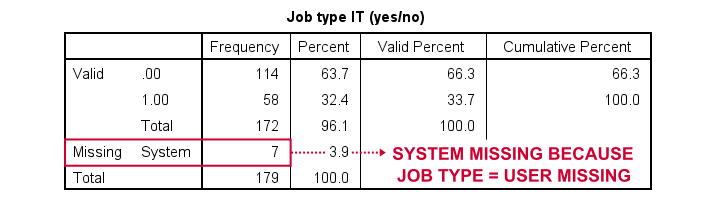## Workarounds for Bugs

The issues discussed in this tutorial are nasty; they may produce incorrect results that easily go unnoticed. A couple of ways to deal with them are the following:

• AUTORECODE string variables into numeric ones and proceed with these numeric counterparts.
• for creating tables and/or charts, exclude cases having string missing values with FILTER or even delete them altogether with SELECT IF.
• for creating new variables, IF and DO IF should suffice for most scenarios.

Right, I guess that should do regarding user missing string values. I hope you found my tutorial helpful. If you've any questions or comments, please throw us a comment below.

•